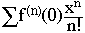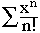Jason Rasmussen (student) Secondary Hello, I have a question regarding power series notation for certain functions within the interval of convergence. I was under the assumption that only functions that can be manipulated into a form resembling geometric series, or derivated/integrated to achieve that form can take on a power series notation. Secondly I thought that taking the derivative of the series to the "nth" order and setting x = a yields the formula for the coefficients as Cn = fn(a)/n!. Putting this back into the standard power series form and setting a = 0 for Maclaurin, f(x) = sigma [fn(0)*xn/n!]. Now, I face the problem understanding how ex = sigma [xn/n!]. I see that the derivative of ex is ex, so that fn(0) = 1 for all n where f(x) = ex. So I understand the 1/n! portion of the series representation, but how do I get the xn which is required by the standard form? Do I do something with the natural log to take the x out of the power? Thank you very much for any feedback. I'm already almost bald. Hi Jason, You have already done everything you need to do. As you said the Maclaurin series representation of a function f(x) isFor the function f(x) = ex, f(n)(0) = 1 for all n and hence the series isCheers, Harley Go to Math Central